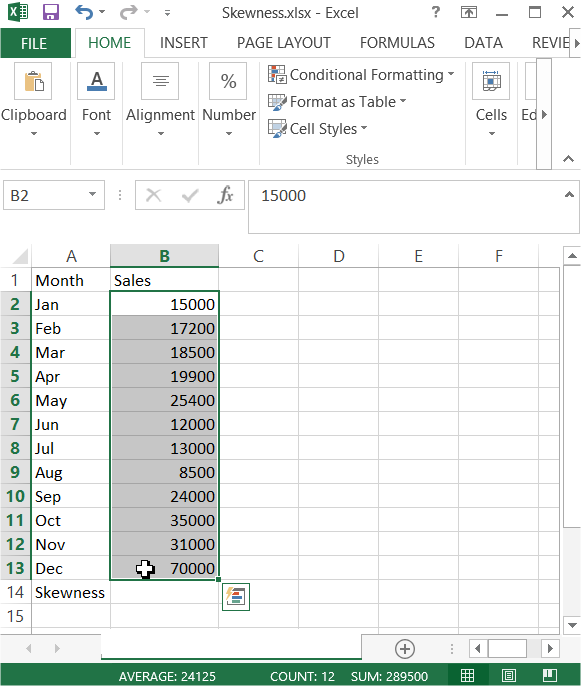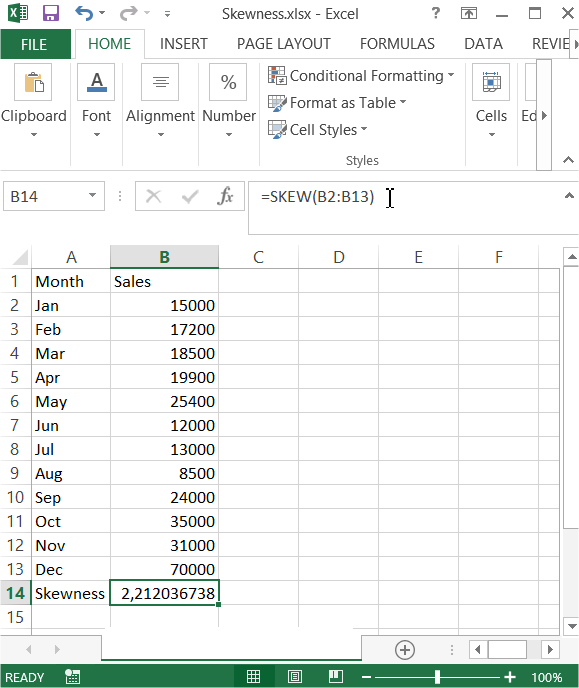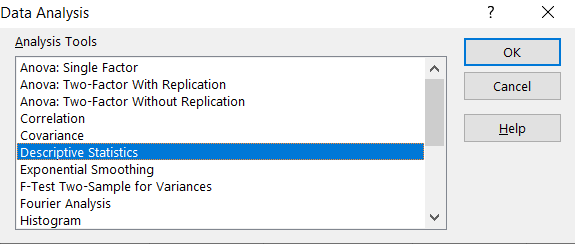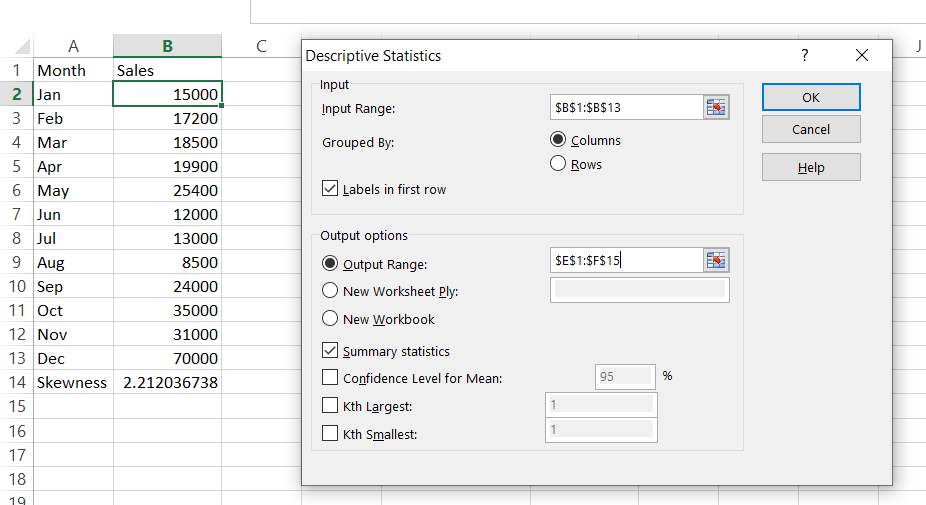## Calculating skewness in Excel

In this Excel tutorial you will teach yourself what the skewness is and how to calculate skewness in Excel application. Skewness is the statistics by which you define asymmetry in the distribution of the analyzed variable. With skewness you’ll see how the results of your variable shape around the average. If their distribution is similar to the normal distribution (skewness equals to 0) or whether you can deal with the distribution positively skewed (skewness > 0) or negatively skewed (skewness <0).To calculate a skewness in Excel please prepare some data table.## Example 1 Calculate Skewness using SKEW function

Excel provides SKEW function. Syntax is:

=SKEW(number1, [number2], …)

In this example formula is: =SKEW(B2:B13)Skewness here is strongly above 0 (skewness > 0) what means that it is a right-sided asymmetry. The conclusion is that for many of months, sales was significantly lower than the average sales.

## Example 2 Calculate Skewness using Data Analysis ToolPak Add-In

First you need to make sure your Data Analysis Toolpak Add-In is installed. Here is the lesson how to install Data Analysis Toolpak Add-In.

Go to Ribbon to the Data tab. Click the Data Analysis button.New window appears. Choose Descriptive Statistics and click OK.Enter the proper parameters:

• Input Range – range of your data (\$B\$1:\$B\$13 in my example)
• Labels in first row – there are labels in my data table
• Output Range – choose where you want to get your skewness calculated (I chose the same Sheet \$E\$1:\$F\$15)
• Summary statistics – make sure it is checkedAnd this is how to see my Statistics summary. Skewness is calculated in row 10. The result is the same as in the Example 1 where we checked how to calculate Skewness using SKEW Excel function.Excel really helps you with statistics. Hopefully now you can see that.

## Template

```Further reading: